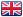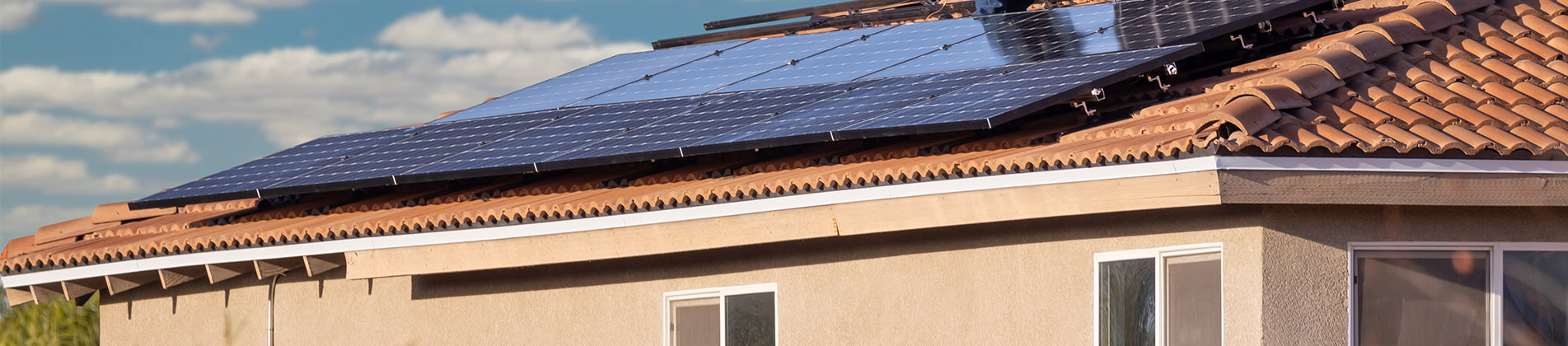enBlog
###### The effect of Tilt Angle On Power Generation Efficiency​
May 19 , 2021
1. What is the influence of the angle between the photovoltaic module and the horizontal plane on the power generation efficiency?
A: Different regions with different latitudes and longitudes have different power generation efficiency. Take Jinan, China as an example (direct south, under the same conditions, 30 degrees is the best inclination angle, without loss of power generation). If the solar module inclination angle is 50 degrees, the power generation loss will be 4 %; the inclination angle is 40 degrees, the power generation loss is 0.9%; the inclination angle is 20 degrees, the power generation loss is 1.3%; the inclination angle is 10 degrees, the power generation loss is 4.6%.

2. Should components of different powers never be connected in series, or can they be connected in series when the power disparity is small? How much allowable power disparity can be connected in series?
A: It is not recommended to do this, because the power of the components is different, the working current is also different. If connected in series, the whole string will follow the smallest component current. The greater the power difference, the greater the current difference.

3. There are also 48pcs pv modules of 450w, 16 one is connected to three channels, 12 one is connected to four channels, and two channels are connected to 14 modules. Is the power generation effect the same?
A: The first one is better. If the designed voltages are all within the MPPT voltage range, then the difference is not big. The only difference is that the line losses produced by different circuits are different.

Categories
New Blog
Tags